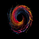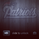14907 просмотров
14907
This long only strategy determines the price of the last fractal top and enters a trade when the price breaks above the last fractal top. The strategy also calculates the average price of the last fractal tops to get the trend direction. The strategy exits the long trade, when the average of the fractal tops is falling (when the trend is lower highs as measured by fractals). And the user can manually set a time delay of this exit condition. The default setting is a long strategy exit always 3 bars after the long entry condition appeared.

In addition as gimmicks the fractals tops can be highlighted (the default is blue) and a line can be drawn based on the fractal tops.This fractal top line is colored by the fractal top average trend in combination with the fractal breakout condition.

This strategy works better on higher time-frames (weekly and monthly), but it also works on the daily and some other time-frames. This strategy does not repaint, no repainting.

P.S. I thank Tradingview user barracuda who helped me with the time based exit condition code. And user RicardoSantos for coding the definition of the fractal top, which he uses in his "Fractals" scripts.

All trading involves high risk; past performance is not necessarily indicative of future results. Hypothetical or simulated performance results have certain inherent limitations. Unlike an actual performance record, simulated results do not represent actual trading. Also, since the trades have not actually been executed, the results may have under- or over-compensated for the impact , if any, of certain market factors, such as lack of liquidity. Simulated trading programs in general are also subject to the fact that they are designed with the benefit of hindsight. No representation is being made that any account will or is likely to achieve profits or losses similar to those shown.
```//@version=2
strategy("Fractal Breakout Strategy (by ChartArt)", shorttitle="CA_-_Fractal_Breakout_Strat", overlay=true)

// ChartArt's Fractal Breakout Strategy
//
// Version 1.0
// Idea by ChartArt on April 24, 2016.
//
// This long only strategy determines the last fractal top
// and enters a trade when the price breaks above the last
// fractal top. The strategy also calculates the average
// price of the last 2 (or 3) fractal tops to get the trend.
//
// The strategy exits the long trade when the average of the
// fractal tops is falling (when the trend is lower highs).
// And the user can manually set a delay of this exit.
//
// In addition the fractals tops can be colored in blue
// and a line can be drawn based on the fractal tops.
// This fractal top line is colored by the fractal trend.
//
// List of my work:
//
//  __             __  ___       __  ___
// /  ` |__|  /\  |__)  |   /\  |__)  |
// \__, |  | /~~\ |  \  |  /~~\ |  \  |
//
//

// input

n_time = input(title='Always exit each trade after this amount of bars later (Most important strategy setting)', defval=3, type=integer)
price = input(hl2,title='Price type to determine the last fractal top and the fractal breakout, the default is (high+low)/2')

// fractal calculation

fractal_top = high > high and high > high and high > high and high > high
fractal_price = valuewhen(fractal_top, price, 1)
use_longer_average = input(true,title='Use Fractal price average of the last 3 fractals instead of the last 2 fractals?')
fractal_average = use_longer_average?(fractal_price + fractal_price + fractal_price ) / 3 : (fractal_price + fractal_price) / 2
fractal_trend = fractal_average > fractal_average
no_repainting = input(true,title='Use the price of the last bar to prevent repainting?')
fractal_breakout = no_repainting?price > fractal_price:price > fractal_price

// highlight fractal tops

show_highlight = input(true,title='Highlight fractal tops in blue and color all other bars in gray?')
highlight = fractal_top?blue:silver
barcolor(show_highlight?highlight:na,offset=-2)
show_fractal_top_line = input(true,title='Draw a colored line based on the fractal tops?')
fractal_top_line = change(fractal_top) != 0 ? price : na
fractal_top_line_color = change(fractal_price) > 0 and fractal_breakout == true ? green : change(fractal_price) < 0 and fractal_breakout == false ? red : blue
plot(show_fractal_top_line?fractal_top_line:na,offset=-2,color=fractal_top_line_color,linewidth=4)

// strategy

trade_exit = fractal_trend[n_time] and fractal_trend == false

strategy.entry('Long', strategy.long)